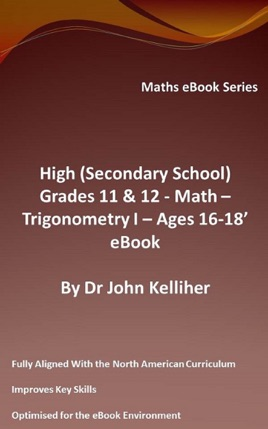• 29,00 kr

## Utgivarens beskrivning

This eBook introduces the trigonometric ratios, the trigonometric ratios of standard triangles, the sine rule, the cosine rule, the formula to calculate the area of a triangle as well as generic trigonometric equations equation of a circle, the graphs of cos x, sin x and tan x, transformed trigonometric graphs as well as the graph and CAST methods of solving trigonometric equations in a given interval along with a selection of questions.